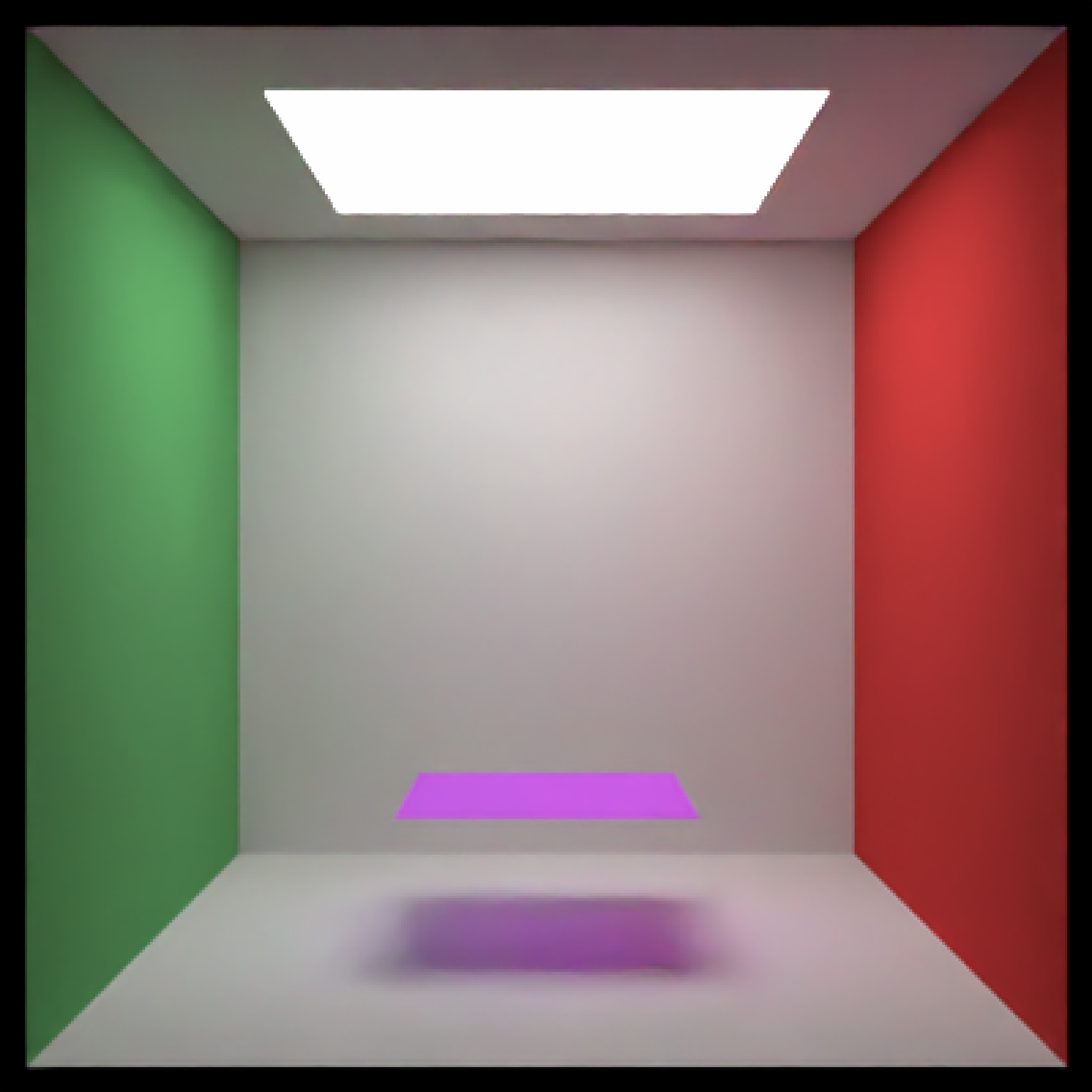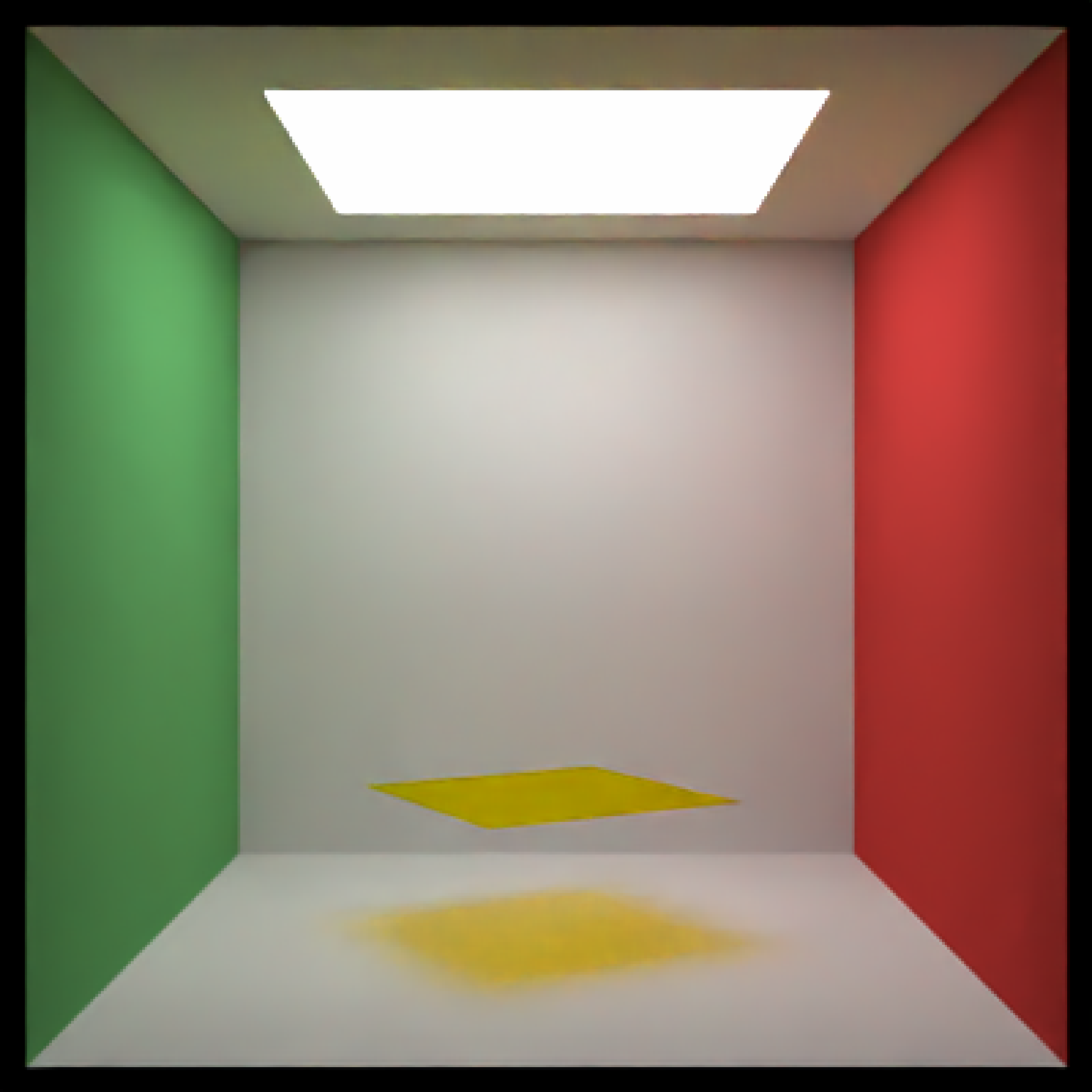Rectangular XZ Plane Object

xz_rect(
x = 0,
xwidth = 1,
z = 0,
zwidth = 1,
y = 0,
material = diffuse(),
angle = c(0, 0, 0),
order_rotation = c(1, 2, 3),
flipped = FALSE,
scale = c(1, 1, 1)
)

## Arguments

x

Default 0. x-coordinate of the center of the rectangle.

xwidth

Default 1. x-width of the rectangle.

z

Default 0. z-coordinate of the center of the rectangle.

zwidth

Default 1. z-width of the rectangle.

y

Default 0. y-coordinate of the center of the rectangle.

material

Default diffuse.The material, called from one of the material functions diffuse, metal, or dielectric.

angle

Default c(0, 0, 0). Angle of rotation around the x, y, and z axes, applied in the order specified in order_rotation.

order_rotation

Default c(1, 2, 3). The order to apply the rotations, referring to "x", "y", and "z".

flipped

Default FALSE. Whether to flip the normals.

scale

Default c(1, 1, 1). Scale transformation in the x, y, and z directions. If this is a single value, number, the object will be scaled uniformly. Note: emissive objects may not currently function correctly when scaled.

## Value

Single row of a tibble describing the XZ plane in the scene.

## Examples

#Generate a purple rectangle in the cornell box.
if(rayrender:::run_documentation()) {
generate_cornell() %>%
add_object(xz_rect(x = 555/2, y = 100, z = 555/2, xwidth = 200, zwidth = 200,
material = diffuse(color = "purple"))) %>%
render_scene(lookfrom = c(278, 278, -800) ,lookat = c(278, 278, 0), fov = 40,
ambient_light = FALSE, samples = 128, parallel = TRUE, clamp_value = 5)
}#Generate a gold plane in the cornell box
if(rayrender:::run_documentation()) {
generate_cornell() %>%
add_object(xz_rect(x = 555/2, y = 100, z = 555/2,
xwidth = 200, zwidth = 200, angle = c(0, 30, 0),
material = metal(color = "gold"))) %>%
render_scene(lookfrom = c(278, 278, -800) ,lookat = c(278, 278, 0), fov = 40,
ambient_light = FALSE, samples = 128, parallel = TRUE, clamp_value = 5)
}